##1. Incircle tangents or the beauty of the equilateral

 The tangent to the incircle of an equilateral triangle at a point P is the trilinear polar of a point at infinity XP, determined by a direction of paralles X.
 The Simson line of P with respect to the incircle is orthogonal to the direction of XP.
 Point P is the isogonal/isotomic conjugate of the point at infinity XP with respect to the medial triangle A''B''C''.
 The isogonal/isotomic conjugate of XP with respect to triangle ABC is point P' anti-homothetic of P in the anti-homothety which interchanges ABC with A''B''C''.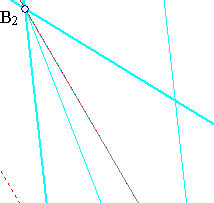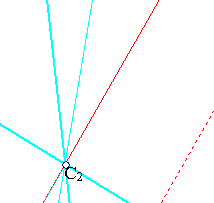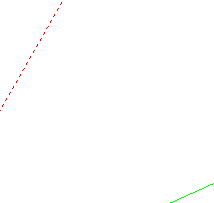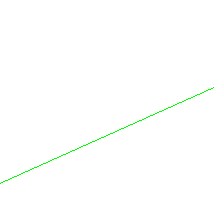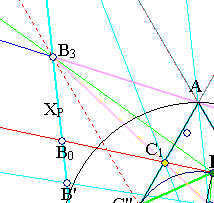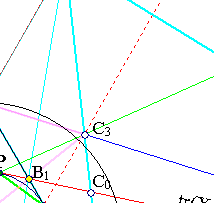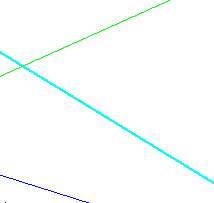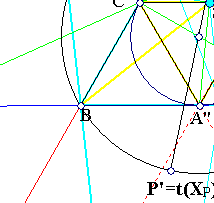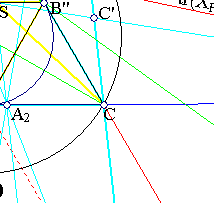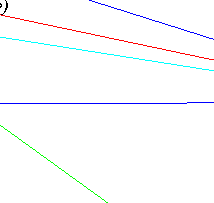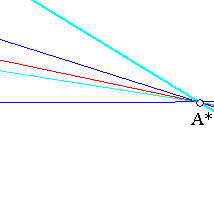Start with point P and the tangent to the incircle of ABC at this point. Quadrilateral PC''A''B'' is inscribed in the circle hence by Pascal's theorem (see PascalOnQuadrangles.html ) the intersection points of opposite sides {B3,C3} and of tangents at opposite vertices {A,A*} are on a line and make a harmonic division.
 Because of the parallels {A''B'',A''C''} to the sides of ABC each of the triangles {AB''C3, B3C''A} is similar to B3A''C3, hence they are similar to each other. It follows that B''C3/AB''=AC''/B3C'' and since {B'',C''} are the middles of sides we get B''C3/B''C=BC''/C''B3, implying that triangles {B3C''B, CB''C3} are similar. Thus lines {CC2,BB2} are parallel.
 Obviously CC2=2CC3 and BB2=2BB3. Hence line B2C2 passes through A*.
 Consider quadrilateral BCB1C1 which circumscribs the incircle and take polars with respect to the incircle. By Brianchon's theorem for quadrangles (see Brianchon2.html ) its diagonals {BB1,CC1} intersect at the same point S at which intersect lines {PA'',C''B''} joining contact points of opposite sides. Since C''P is the polar of C1 and passes through C3, the polar of C3 will pass also through C1. Analogously it will pass through C. Hence C1C coinsides with the polar of C3 and will pass through B3. Analogously BB1 passes through C3. Thus B3C3 is the polar of S.
 The bundle of lines B(C,C3,C2,B3) is harmonic (since CC3=C3C2). Hence defines on AC a harmonic division. This implies that B1 is the harmonic conjugate of B2 with respect to {A,C}. Analogously C1 is the harmonic conjugate of C2 with respect to {A,B}. This implies:
(i) S is the tripole of line B2C2.
(ii) Lines {B1C2,C1B2} intersect at a point A2 on line BC.
(iii) Line AA3 is parallel to lines BB3 and CC3.
 Since (B1,B2,A,C) and (C1,C2,A,B) are harmonic divisions line B1C1 is the tripolar of the point at infinity determined by the parallel lines {BB2,CC2} (apply the definition of tripolar).

Remark The reasoning here is to work from point P on the circumcircle and the tangent at P up to the tripole of this line with respect to ABC, which is the point at infinity determined by the direction BB2.
In the file HyperbolaPropertyParallels.html the same result is obtained in the reverse way, by starting from the point at infinity and landing down to P.

The statement on the Simson line is immediate and follows by an angle chasing argument indicated in the figure below. The figure is a magnification of the previous one. The small circle passes through points P and B'' and the two projections of P on the sides of A''B''C''. It results by the definition of the Simson line of P with respect to A''B''C''. The angles indicated at P are equal because angle(A''PB'') is 60 degrees as is also the angle of the cyclic quadrilateral at P opposite to B''.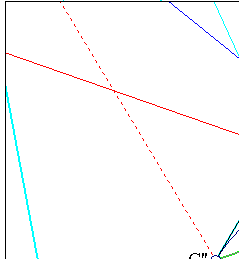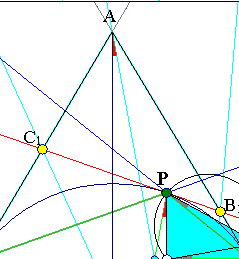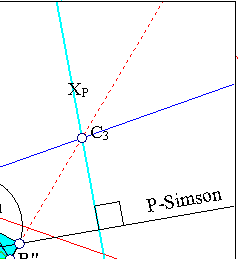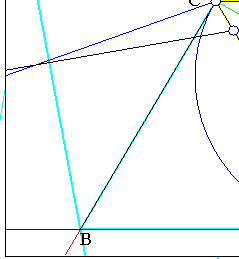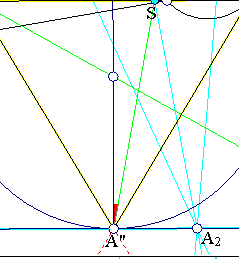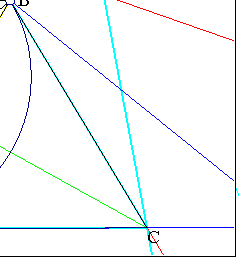The fact that P is the isogonal conjugate of the point at infinity XP with respect to triangle A''B''C'' follows from the discussion on isogonal conjugates of points of the circumcircle in IsogonalOfCircumcircle.html .
The statement on the anti-homothety F interchanging ABC and A''B''C'' follows from the previous result and the fact that t(P') = t(F(P)) = F(t(P)) = F(XP) = XP. Last equation being the obvious invariance of the direction of parallels by the anti-homothety F. The fact that t*F = F*t (* denoting the composition of maps) is discussed in some extension in IsogonalGeneralized.html .

##2. Two perspective triangles

 Triangle SB3C3 is self-polar with respect to the incircle of ABC, perspective to it and its side-lines pass through the vertices of ABC.
 The perspector is the point at infinity XP and the line of perspectivity ( Desargues.html ) is the tangent PA* at P.
 The tripole of line B1C1 with respect to triangle SB3C3 is the same point at infinity determined by XP.
 Segments {A0A2,B0B2,C0C2} are correspondingly the double of {AA0,BB0,CC0}. Points {A0,B0,C0} denote correspondingly the intersection points of the parallel lines {AA2,BB2,CC2} with the tangent PA*.
 Define the affinity H of the plane by the properties :
(i) H fixes the points of line PA*.
(ii) Every point Q is mapped to Q'=H(Q) such that QQ' is parallel to XP and line PA* divides QQ' in ratio 2, i.e. the intersection point Q0 of QQ' with PA* satisfies QQ0/Q0Q'=2.
The affinity H can be interpreted as a perspectivity with center at the point at infinity defined through XP, axis the line PA* and homology coefficient k=-0.5. It maps triangle ABC to triangle SB3C3.

[1,2] are consequences of the discussion in section-1. Because of the harmonicity of tetrads (S,C3,B,B1) etc. the tripole of line B1C1 with respect to triangle SB3C3 is also the point at infinity determined by XP. This proves .  is a consequence of  of section-1.  is a consequence of .

Remark These facts generalize to a property for self-polar triangles with respect to a conic. See the relative discussion in Autopolar2.html .

Autopolar2.html
Brianchon2.html
CircumconicsTangents.html
Desargues.html
HyperbolaPropertyParallels.html
HyperbolaPropertyParallels2.html
IncircleTangents2.html